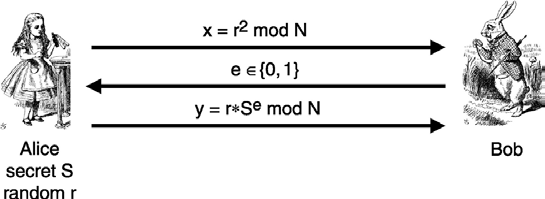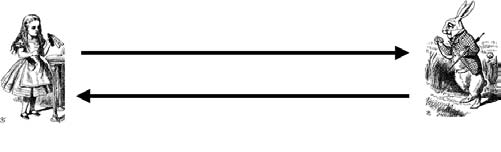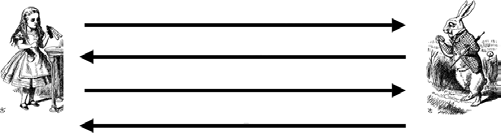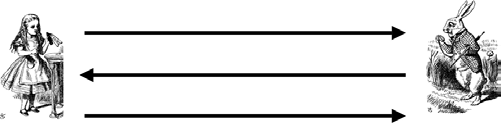Rating : ⭐⭐⭐⭐⭐
Price : \$10.99
Language:EN
Pages: 5

# Your protocols must use timestamp and encrypt and sign

228 SIMPLE AUTHENTICATION PROTOCOLSThe Fiat-Shamir protocol, as illustrated in Figure 9.32, proceeds as follows. Alice selects a random r, and she sends x = r2mod N to Bob. Bob then chooses a random value e ∈ {0, 1}, which he sends to Alice. In the third message, Alice responds with y = rSemod N and Bob then verifies that

y2= xvemod N

and equation 9.1 becomes If e = 0 in Fiat-Shamir, thenAlice responds in the third message with y = r mod N

y2= r2= x mod N.

Suppose Trudy expects Bob to send the challenge e = 0 in message two. Then Trudy can send x = r2mod N in message one and y = r mod N in message three. In other words, Trudy simply follows the protocol in this case, since she does not need to know the secret S.

On the other hand, if Trudy expects Bob to send e = 1, then she can send x = r2v−1mod N in message one and y = r mod N in message three. Then Bob will com-pute y2= r2and xve= r2v−1v = r2and he will find that equation 9.1 holds, and he will accept the result as valid. But if Bob chooses e ∈ {0, 1} at random (as required by the protocol), then Trudy can only fool Bob with probability1 2. And, as with Bob’s Cave, after n iterations, the probability that Trudy can fool Bob is only (1 2)n.

Asignificantadvantageofzeroknowledgeproofsisthattheyallowforauthentication with anonymity. In Fiat-Shamir, both sides must know the public value v, but there is nothing in v that identifies Alice, and there is nothing in the messages that are passed that must identify Alice. This is a significant advantage that has led Microsoft to include support for zero knowledge proofs in its “next generation secure computing base,” or

230 SIMPLE AUTHENTICATION PROTOCOLS

• What delay is tolerable?

• What type of crypto is supported—public key, symmetric key, or hash functions?• Is mutual authentication required?

9.7 SUMMARY

In this chapter we discussed several different ways to authenticate and establish a session key over a network. We can accomplish these feats using symmetric keys, public keys, or hash functions. We also learned how to achieve perfect forward secrecy, and we considered the benefits (and potential drawbacks) of using timestamps.

3. Provide a way to achieve perfect forward secrecy that does not use Diffie-Hellman.

4. The insecure protocol in Figure 9.24 was modified to be secure in Figure 9.26. Find two other ways to slightly modify the protocol in Figure 9.24 so that the resulting protocol is secure. Your protocols must use a timestamp and “encrypt and sign.”

“I’m Alice”, R

E(R, KAB)

8. What is the primary advantage of using timestamps in an authentication protocol. What is the primary disadvantage of using timestamps?

9. Consider the following protocol, where K = h(S, RA, RB) and CLNT and SRVR are constants:

a. Does Alice authenticate Bob? Why or why not?

b. Does Bob authenticate Alice? Why or why not?

Alice E(SRVR, K) Bob232 SIMPLE AUTHENTICATION PROTOCOLS

[T + 1]Bob, {K}Alice

Alice Bob

[RB]Bob

{S}Bob, E(RA, K)

{RA, RB}Alice

E(RB, K)

How It Works